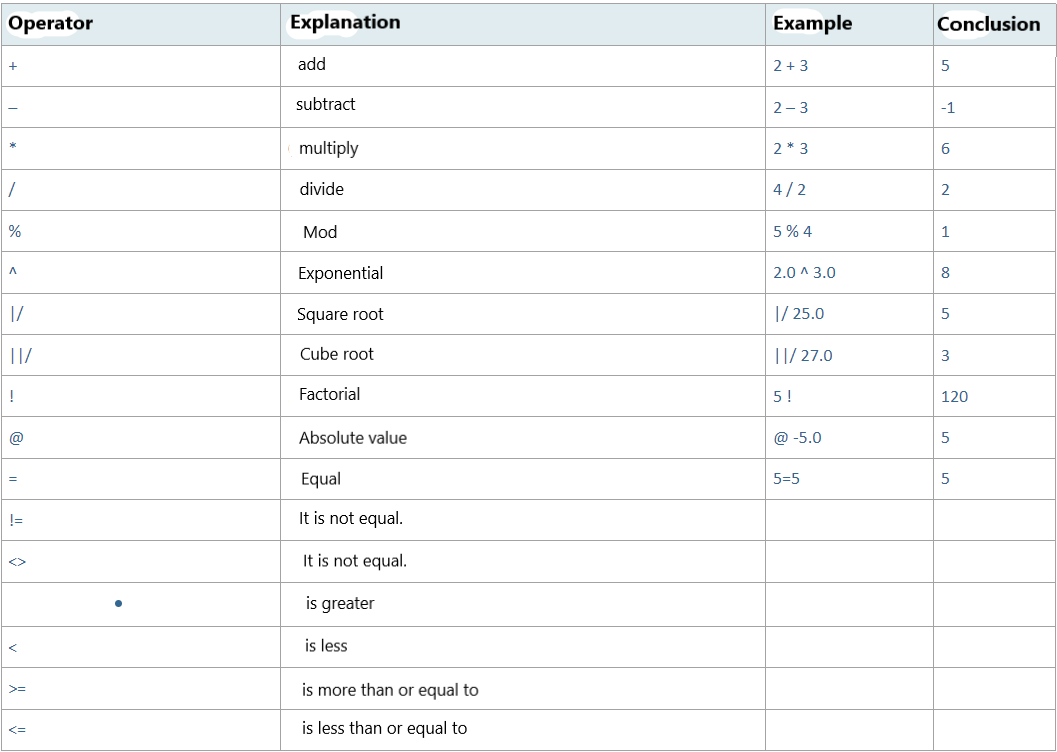Thursday , June 8 2023

# PostgreSQL Arithmetic Operators

In PostgreSQL, we can easily perform operations such as addition, subtraction, division, multiplication, square root or factorial between numbers or two columns.

#### Operator IconsLet’s make an example using some operators:The vize and final columns in our student table have integer data type, let’s collect them.In the example above, we collected the vize and final grades of 3 students and saw which student they belonged to, but how would we do it if we wanted 40% of the vize to take 70% of the final and say the total passing grade?

Let’s do this together.In the example above, we first multiplied the values in the vize by 0.40 and got 40%, then we multiplied the final column by 0.70 to get 70% and summed the result from the two columns.We found the factorial value of the value specified in the above example.